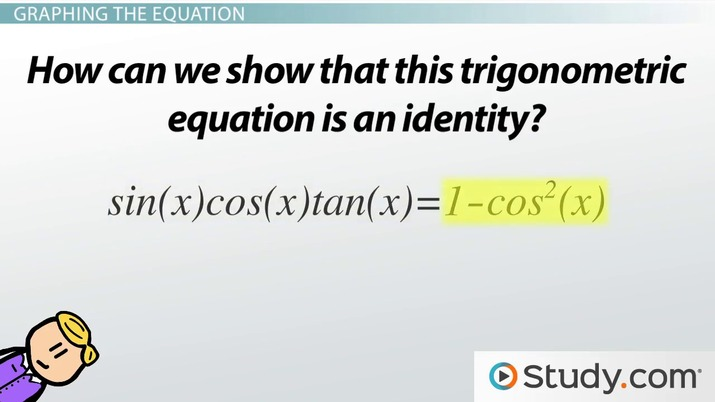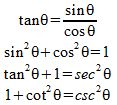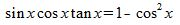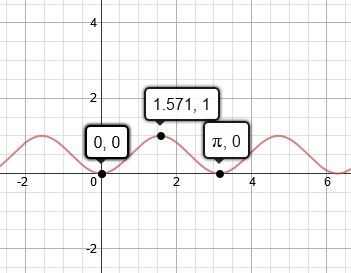Using Graphs to Determine Trigonometric Identity

Lesson Transcript
Instructor: Yuanxin (Amy) Yang Alcocer

Amy has a master's degree in secondary education and has been teaching math for over 9 years. Amy has worked with students at all levels from those with special needs to those that are gifted.

A trigonometric identity refers to an equation that is consistently true, regardless of what value is applied to the variable. Learn more about this concept through example equations that are graphed, and discover how to demonstrate whether or not a trigonometric equation is an identity. Updated: 10/27/2021

Trigonometric Identity

In this video lesson, you will learn what to look for in a graph to determine whether a particular equation is a trigonometric identity or not. But first, let's define trigonometric identity. A trigonometric identity is any trigonometric equation that is always true for all values of the variable. What does this mean?

This means that if you see an equation and you can say for certain that this equation is always true and you can use the equation with confidence, then this trigonometric equation is a trigonometric identity. Remember identity means the same. Think of the related word 'identical.' You can also think of identity as meaning 'self.' Like when someone asks you, 'What is your identity?' You answer with the kind of person you are or you show your identification card, your identity card.An error occurred trying to load this video.

Try refreshing the page, or contact customer support.

Coming up next: Solving a Trigonometric Equation Graphically

You're on a roll. Keep up the good work!

Replay
Your next lesson will play in 10 seconds
• 0:01 Trigonometric Identity
• 0:53 Trigonometric Equations
• 2:17 Graphing the Equation
• 4:04 Lesson Summary
Save Save

Want to watch this again later?

Timeline
Autoplay
Autoplay
Speed Speed

Trigonometric Equations

So, what kinds of equations can you expect to see as we look at trigonometric equations? In math, we already have some trigonometric equations that we know are true. Once we know that a certain trigonometric equation is true, then we can call it a trigonometric identity. For example, these trigonometric equations have been known as trigonometric identities for many, many years:We use these trig identities to help us solve more complicated trig problems. For example, if we see sine squared plus cosine squared inside a larger problem, we can substitute 1 for that part of the problem, thus making the problem that much simpler and easier to deal with.

Let's take a look at a trigonometric equation that we have been given:Many times, when we are working with trigonometric equations, you will see a variable such as x instead of theta. The letter or symbol that is used to represent our variable doesn't matter. As long as you know which letter is your variable, that is all that matters. So, here x is our variable. So, the question now is how can we show that this trigonometric equation is an identity?

Graphing the Equation

We can do that by graphing each side of our equation. So, what we do is we first graph sin (x) cos (x) tan (x), then we graph 1 - cos^2 (x) to see if they are equal. We can use a graphing calculator to do this or any other graphical method that is easy for you.

Why don't we go ahead and graph each side.

First, the left side, sin (x) cos (x) tan (x). We get this graph:To unlock this lesson you must be a Study.com Member.

Register to view this lesson

Are you a student or a teacher?